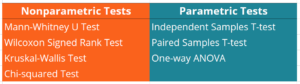# Nonparametric Tests

Methods of statistical analysis that do not require a distribution to meet the required assumptions to be analyzed

## What are Nonparametric Tests?

In statistics, nonparametric tests are methods of statistical analysis that do not require a distribution to meet the required assumptions to be analyzed (especially if the data is not normally distributed). Due to such a reason, they are sometimes referred to as distribution-free tests. Nonparametric tests serve as an alternative to parametric tests such as T-test or ANOVA that can be employed only if the underlying data satisfies certain criteria and assumptions.Note that nonparametric tests are used as an alternative method to parametric tests, not as their substitutes. In other words, if the data meets the required assumptions for performing the parametric tests, the relevant parametric test must be applied.

In addition, in some cases, even if the data do not meet the necessary assumptions but the sample size of the data is large enough, we can still apply the parametric tests instead of the nonparametric tests.

### Reasons to Use Nonparametric Tests

In order to achieve the correct results from the statistical analysis, we should know the situations in which the application of nonparametric tests is appropriate. The main reasons to apply the nonparametric test include the following:

#### 1. The underlying data do not meet the assumptions about the population sample

Generally, the application of parametric tests requires various assumptions to be satisfied. For example, the data should follow a normal distribution and the population variance is homogeneous. However, some data samples may show skewed distributions.

The skewness makes the parametric tests less powerful because the mean is no longer the best measure of central tendency because it is strongly affected by the extreme values. At the same time, nonparametric tests work well with skewed distributions and distributions that are better represented by the median.

#### 2. The population sample size is too small

The sample size is an important assumption in selecting the appropriate statistical method. If a sample size is reasonably large, the applicable parametric test can be used. However, if a sample size is too small, it is possible that you may not be able to validate the distribution of the data. Thus, the application of nonparametric tests is the only suitable option.

#### 3. The analyzed data is ordinal or nominal

Unlike parametric tests that can work only with the continuous data, nonparametric tests can be applied to other data types such as ordinal or nominal data. For such types of variables, the nonparametric tests are the only appropriate solution.

### Types of Nonparametric Tests

Nonparametric tests include numerous methods and models. Below are the most common nonparametric tests and their corresponding parametric counterparts:

#### 1. Mann-Whitney U Test

The Mann-Whitney U Test is a nonparametric version of the independent samples t-test. The test primarily deals with two independent samples that contain ordinal data.

#### 2. Wilcoxon Signed Rank Test

The Wilcoxon Signed Rank Test is a nonparametric counterpart of the paired samples t-test. The test compares two dependent samples with ordinal data.

#### 3. The Kruskal-Wallis Test

The Kruskal-Wallis Test is a nonparametric alternative to the one-way ANOVA. The Kruskal-Wallis test is used to compare more than two independent groups with ordinal data.

#### 4. Chi-squared (x2) Test

The Chi-squared Test is a parametric test that determines the significance of the difference between the expected and observed frequencies in one or more categories. The test is applied to categorical data.